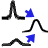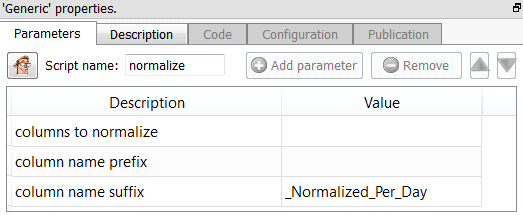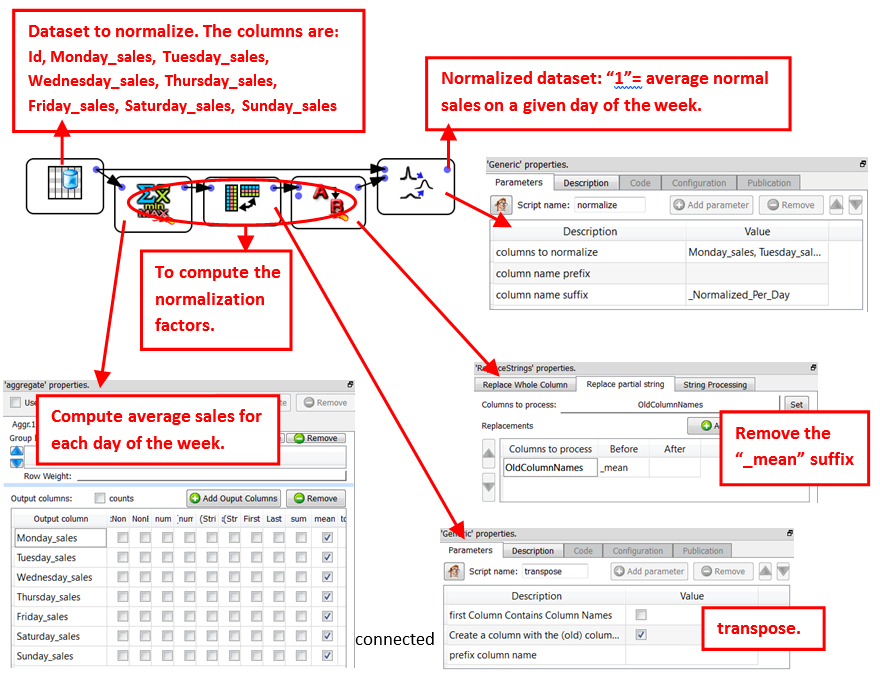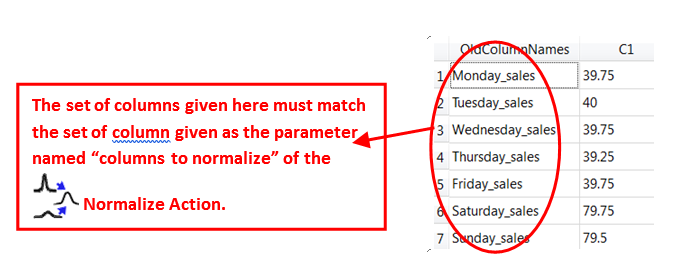﻿ 5. Detailed description of the Actions > 5.7. Data Mining > 5.7.8. Normalize

# 5.7.8. Normalize

Icon:Function: normalize

Property window:Short description:

Apply a normalization factor to some columns based on their name.

Long Description:

Let’s assume that you have a table where each row represents a week and the columns contain the sales for each different day of the week. We want to normalize this dataset so that the value “1” represents the average normal sales on a given day of the week. Such kind of data transformation is useful when you want to create a predictive model that takes into account the “seasonality effects” (more precisely here: The seasonality effect of the day of the week). We’ll have:The pin 0 of theNormalize Action is connected to the dataset to normalize.The pin 1 of theNormalize Action is connected to a table with two columns. The first column contains the name of the columns to normalize. The second column contains the normalization factors. For example, in the above Anatella graph, we have: